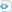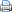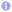# New TRNG release

A new version of TRNG (Tina’s Random Number Generator Library) has been released. TRNG may be utilized in sequential as well as in parallel Monte Carlo simulations. It does not depend on a specific parallelization technique, e.g., POSIX threads, MPI or others. As an outstanding new feature of the latest TRNG release 4.11 it also supports CUDA. See TRNG documentation for details. If you need a modern C++ random number generator library for sequential or parallel Monte Carlo then check out TRNG 4.11.

The following piece of C++ code exemplifies how to use TRNG in a CUDA program. See TRNG documentation for details.

 Source code#include <cstdlib>
#include <iostream>
#include <trng/yarn5s.hpp>
#include <trng/uniform01_dist.hpp>

__global__
void parallel_pi(long samples, long *in, trng::yarn5s r) {
long size=blockDim.x;
trng::uniform01_dist<float> u;      // random number distribution
in[rank]=0;                         // local number of points in circle
for (long i=rank*samples/size; i<(rank+1)*samples/size; ++i) {
float x=u(r), y=u(r);             // choose random x- and y-coordinates
if (x*x+y*y<=1)                   // is point in circle?
}
}

int main(int argc, char *argv[]) {
const long samples=1000000l;             // total number of points in square
const int size=128;                      // number of threads
long *in_device;
cudaMalloc(&in_device, size*sizeof(*in_device));
trng::yarn5s r;
// start parallel Monte Carlo
parallel_pi<<<1, size>>>(samples, in_device, r);
// gather results
long *in=new long[size];
cudaMemcpy(in, in_device, size*sizeof(*in), cudaMemcpyDeviceToHost);
long sum=0;
for (int rank=0; rank<size; ++rank)
sum+=in[rank];
// print result
std::cout << "pi = " << 4.0*sum/samples << std::endl;
return EXIT_SUCCESS;
}